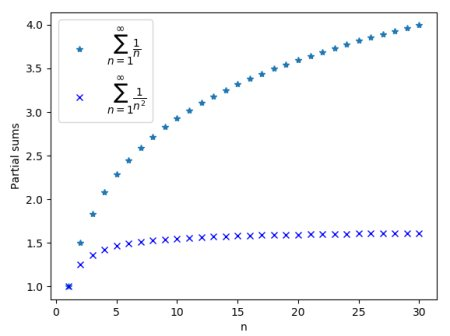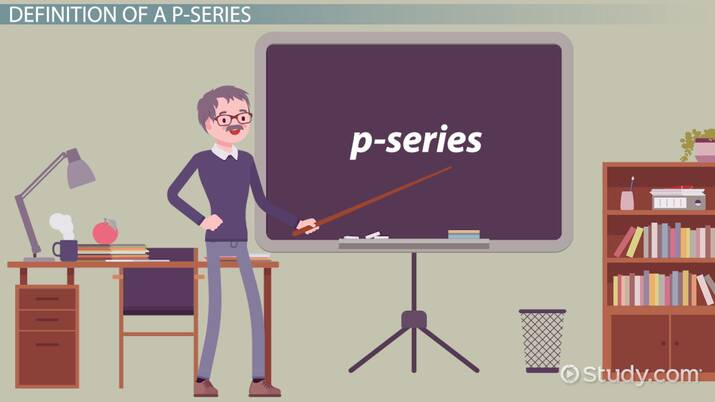# The P-Series Test For Convergence

Mark Lewis, Cameron Smith
• Author
Mark Lewis

Mark has taught college and university mathematics for over 8 years. He has a PhD in mathematics from Queen's University and previously majored in math and physics at the University of Victoria. He has extensive experience as a private tutor.

• Instructor
Cameron Smith

Cameron has a Master's Degree in education and has taught HS Math for over 25 years.

Read what a p-series is and some examples of it. Learn the harmonic series and the p-series test for convergence to prove if it is convergent or divergent. Updated: 01/22/2022

Show

## P-Series

A {eq}p {/eq}-series is any one of a family of infinite series whose terms are reciprocal powers of natural numbers. The parameter {eq}p\in\mathbb{R} {/eq} specifies the power, which defines the series.

Any {eq}p {/eq}-series can be expressed in summation notation using the following general formula:

$$\displaystyle \sum_{n=1}^\infty \dfrac{1}{n^p}$$

For example, if we choose the power {eq}p=2 {/eq}, the corresponding {eq}p {/eq}-series is the sum of all reciprocals of perfect square numbers:

$$\begin{eqnarray} \sum_{n=1}^\infty \dfrac{1}{n^2} &=& \dfrac{1}{1^2} + \dfrac{1}{2^2} + \dfrac{1}{3^2} + \dfrac{1}{4^2} + \ldots \\ &=& 1 + \frac{1}{4} + \frac{1}{9} + \frac{1}{16} + \ldots \end{eqnarray}$$

{eq}p {/eq}-series in calculus has applications because series are closely connected to definite integrals, which compute the area underneath a function {eq}f(x) {/eq}. In particular, the convergence of some integrals can be determined by comparing the convergence of a corresponding series, which could be a {eq}p {/eq}-series.

### Harmonic Series

The series which consists of the sum of the reciprocals of all natural numbers is known as the harmonic series. Its general formula, and calculation term-by-term, are

$$\displaystyle \sum_{n=1}^\infty \dfrac{1}{n } = 1 + \dfrac{1}{2} + \dfrac{1}{3} + \dfrac{1}{4} + \ldots$$

We can notice that this is simply a special case of a {eq}p {/eq}-series with the power {eq}p=1 {/eq}. This simple member of the family turns out to play a key role in determining the {eq}p {/eq}-series convergence of divergence, as we'll see momentarily, when we discuss the {eq}p {/eq}-series test.

### Sum of P-Series

There is no easy way to calculate the exact sum of a given {eq}p {/eq}-series, though the value of some specific series have been determined. For example the exact value of the series for {eq}p=2 {/eq} is

$$\displaystyle \sum_{n=1}^\infty \dfrac{1}{n^2} = \dfrac{ \pi^2}{6} \approx 1.65$$

The {eq}p {/eq}-series test can at least determine whether or not a particular series converges at all, and an approximate value can then be found by adding up terms in the sum.An error occurred trying to load this video.

Try refreshing the page, or contact customer support.

Coming up next: Harmonic Series in Math: Definition & Formula

### You're on a roll. Keep up the good work!

Replay
Your next lesson will play in 10 seconds
• 0:04 Definition of a p-Series
• 1:00 Examples of p-Series
• 1:57 Why Can't p Be Negative?
• 2:49 p-Series in Disguise
• 3:11 Lesson Summary
Save Save

Want to watch this again later?

Timeline
Autoplay
Autoplay
Speed Speed

## P-Series Test for Convergence

Whether or not a {eq}p {/eq}-series converges to a specific value can be determined using the very simple {eq}p {/eq}-series test for convergence. The test states that the series

$$\displaystyle \sum_{n=1}^\infty \dfrac{1}{n^p}$$

will converge if {eq}p>1 {/eq}, otherwise it will diverge.

This rule for {eq}p {/eq} series is consistent with our earlier example with power {eq}p =2 > 1 {/eq}, which we saw was a convergent series.

## P-Series Examples

Lets consider several {eq}p {/eq}-series examples, and determine their convergence using the {eq}p {/eq}-series test.

### Example 1

Lets begin by determining the convergence of the harmonic series. Since this is a {eq}p {/eq}-series with power {eq}p=1 {/eq}, the {eq}p {/eq}-series test tells us that the harmonic series diverges.

The terms of the harmonic series quickly become very small numbers. If you try adding them up, it may seem that the sum is going to converge. Nevertheless, as more terms are added the sum will grow very large - but very slowly!

According to the {eq}p {/eq}-series rule, the harmonic series is the dividing line between convergent and divergent {eq}p {/eq}-series, since the series do converge for any power strictly above {eq}p=1 {/eq}.

To unlock this lesson you must be a Study.com Member.

#### What is the P series formula?

All p-series can be represented as the infinite sum of the reciprocal powers of the natural numbers. The general formula is the sum of 1/n^p for n between 1 and infinity.

#### What is a convergent p series?

An infinite series converges if the limit of its sum approaches a specific finite value. p-series converge when the power appearing in the denominator of each term satisfies p>1.

#### What is the P series test?

The p-series test can be used to determine if a p-series converges or diverges. It converges if, and only if, the power satisfies p>1.

#### How do you know if a series is P series?

The terms in a p-series are reciprocal powers of natural numbers. This means they have the form 1/n^p for some fixed power p.

### Register to view this lesson

Are you a student or a teacher?